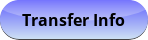Sep 21, 2023
2023-2024 College Catalog
 Select a Catalog 2023-2024 College Catalog 2022-2023 College Catalog [ARCHIVED CATALOG] 2021-2022 College Catalog [ARCHIVED CATALOG] 2020-2021 College Catalog [ARCHIVED CATALOG] 2019-2020 College Catalog [ARCHIVED CATALOG] *** DRAFT 2024-2025 College Catalog DRAFT *** [ARCHIVED CATALOG]
 HELP 2023-2024 College Catalog Print-Friendly Page (opens a new window) Add to Portfolio (opens a new window)

# MAT 106 - Elementary Data Analysis with Spreadsheets

3 Credits, 3 Contact Hours
3 lecture periods 0 lab periods

Introduction to statistics. Includes the collection and presentation of data, statistical measures, algebra topics, Excel topics, and data analysis topics.

Gen-Ed: Meets CTE - M&S.Course Learning Outcomes
1. Analyze data including central tendencies and variation.
2. Demonstrate knowledge of various data models including line of best fit and normal distribution.
3. Describe and display data using a spreadsheet.

Performance Objectives:
1. Distinguish between populations and samples, compare different sampling methods, and describe possible sample biases.
2. Represent data in various graphical forms including histograms, pie charts and tables, and interpret information presented in graphical form.
3. Set up Excel spreadsheets using formulas that reference other cells, use mathematical operations including integer exponents, and require application of the order of operations; create charts and graphs in Excel.
4. Determine measures of central tendency of univariate data including means, modes, and quartile ranges.
5. Determine measures of variability of central tendency of univariate data including mean absolute deviation and standard deviation.
6. Define bivariate data; determine the correlation coefficient for bivariate data, and discuss issues of correlation and causation.
7. Use least squares regression to determine the equation of the “line of best fit”, use the equation to estimate other data points, and discuss predictive value of regression lines.
8. Discuss the impact of outliers for both univariate and bivariate data.
9. Use the normal model, standard normal model, and Z-score to measure and compare data from different distribution; extend these ideas to compute a confidence interval when comparing sample and population statistics.

Outline:
1. Collection of Data
1. Define population and sample
2. Sampling techniques
3. Sampling bias
2. Presentation of Data
1. Tables and graphs
2. Interpretation of graphical data
3. Excel Topics
1. Review of basic operations
2. Chart wizard
3. Data analysis tool pack
4. Measures of Central Tendency of Univariate Data
1. Mean, median and mode
2. Quartile ranges
5. Variability of Central Tendency
1. Mean absolute deviation
2. Standard deviation
6. Bivariate Data Measures
1. Analysis of bivariate data
2. Correlation coefficient
3. Causation versus correlation
7. Linear Regression
1. Least squares regression
2. Use regression line to estimate other points
3. Discuss predictive abilities of regression
8. Outliers
1. Univariate
2. Bivariate
9. Normal Curve
1. Normal model and standard normal model
2. Z score
3. Confidence intervals

Effective Term: